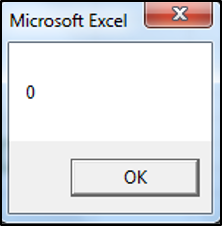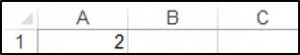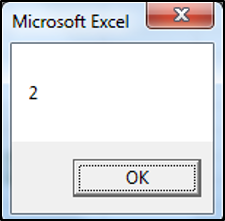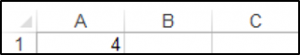### VBA Static Variable

A VBA Static variable retains its value even when the procedure ends.

### Declaring a Static Variable

You declare a Static variable using the Static keyword as shown below:

```Sub DeclaringAStaticVariable()

Static myVariable As Integer
MsgBox myVariable
myVariable = myVariable + 2

Range("A1").Value = myVariable

End Sub```

The first time you press F5, you get a Message box showing the initial value of myVariable which is 0. The value of 2 is placed in cell A1.If you press F5 again immediately afterwards, you get the following Message box showing the value of myVariable which is 2, and the value of 4, placed in your worksheet in cell A1.As you can see the variable is retaining its value even as the Sub Procedure ends, if you used the Dim keyword instead of the Static keyword to declare the variable, the Message Box would always show the value of 0. Also, the result in cell A1, would be 2, every time you clicked F5. Since the variable would not retain its value once the Sub Procedure ended.